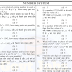## Number System Questions for Bank Exams PDF: Tips, Tricks, and Sample Questions

Number system questions are an integral part of the bank exams. It is one of the most scoring topics in the bank exams. If you are preparing for bank exams, you need to be proficient in number system concepts. In this article, we have compiled some important tips, tricks, and sample questions for number system that will help you in preparing for the bank exams. We have also provided a PDF download link for number system questions for bank exams.

Tips and Tricks for Number System Questions

1. Learn the basics: The first step towards mastering number system questions is to learn the basics. You should be well-versed with the types of numbers, properties of numbers, and the different operations involved in number system.

2. Practice regularly: Number system questions require a lot of practice. The more you practice, the better you get. Make it a habit to solve at least 10-15 questions on number system every day.

3. Use shortcuts: There are many shortcuts that can be used to solve number system questions quickly. Learn and practice these shortcuts to save time and improve your accuracy.

4. Memorize squares, cubes, and roots: It is important to memorize squares, cubes, and roots of numbers up to a certain limit. This will help you in solving number system questions quickly.

Sample Questions for Number System

1. Find the smallest number which when divided by 5, 6, 8, and 9 leaves a remainder of 1.

a) 289 b) 361 c) 481 d) 721

1. What is the remainder when 3^99 is divided by 7?

a) 1 b) 2 c) 3 d) 4

1. Find the HCF of 96 and 404.

a) 2 b) 4 c) 8 d) 16

1. Simplify: (3^4 × 5^3) ÷ (3^2 × 5^2)

a) 15 b) 25 c) 75 d) 125

1. What is the value of (√2 + √3)^2?

a) 5 b) 7 c) 11 d) 13

Conclusion

Number system questions are an important part of the bank exams. It requires a lot of practice and understanding of the concepts. We hope that the tips, tricks, and sample questions provided in this article will help you in preparing for the bank exams. Keep practicing and all the best for your exams!

Number System Questions for Bank Exams PDFReviewed by SSC NOTES on September 25, 2023 Rating: 5